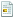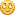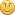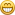January 27, 2021, 10:07:53 am

## News:

Own IWBasic 2.x ? -----> Get your free upgrade to 3.x now.........

## Rounding to an Arbitrary number of Significant Digits.

Started by GWS, May 13, 2013, 02:00:43 am

0 Members and 1 Guest are viewing this topic.

#### GWS

#####May 13, 2013, 02:00:43 am
Hi,

Here's a little problem I came across while working on a current project - how to round a number to a given number of significant digits.

That should be easy, I thought.  For example I wanted a number like 12.1257 to return 12.1

After screwing my brains into a knot, I got some clunky code working (which I won't for shame reveal), except to say, it involved a lot of string handling of the Val() to Str\$() to Val() type.How difficult could a simple operation be?

Fortunately, my daughter is an expert Googler, found this discussion in some java - C hieroglyphics, which I think I've managed to translate into the much easier to read Basic code.

Anyway, here's the test program I've concocted, to take any positive, non-zero number, and re-map it to a given number of significant figures.  I was interested in 2 or 3 figures for my application.Code Select
`' Rounding to a given number of significant figures ..' GWS - 2013openconsoleclsautodefine = "off"setprecision 5def n:intdef x,r:doubledeclare Round(value:double,nfigs:int)' some test values ..x = 12.1257'x = 4855600'x = 2008'x = 0.0681'x = .55578'x = 1.50666'x = 27460'x = 635.89r = Round(x,3)print "rounded = ",rdo:until inkey\$<>""closeconsoleendsub Round(value,nfigs)def ret,d:double' routine to round a value to 'n' significant figures .. if (value = 0) ret = 0 else d = ceil(log10(value)) n = -(d - nfigs) ret = int(value * 10^n + 0.5) * 10^-n endifreturn ret`

Look at that lovely little subroutine - is that smart or what?
I'm still not sure I understand how it does it - but it works!http://stackoverflow.com/questions/202302/rounding-to-an-arbitrary-number-of-significant-digits

All the best,Graham
Tomorrow may be too late ..

#### Kian

#####May 13, 2013, 12:55:39 pm #1
Hi Graham,

QuoteI'm still not sure I understand how it does it

Code Select
`if (value = 0)`
This ensures that '0' is not passed to the logarithm function as log(0) is undefined.

Code Select
`d = ceil(log10(value))`
This returns how many digits there are before the decimal point, using the 'ceil' function to ensure rounding up.
(e.g. log(12.345) is 1.0914911 because it is between 10^1 and 10^2, and this is rounded up to 2)
Values below zero give a negative result for this.

Code Select
`n = -(d - nfigs)`
('nfigs-d' would probably save a cycle or two)
This calculates how many columns to shift the digits for the purposes of rounding.
(e.g. to find 12.345 to 3 s.f. it should be shifted 1 column to the left to round after the 3)

Code Select
`ret = int(value * 10^n + 0.5) * 10^-n`
The 'value * 10^n' performs the shift, i.e. turns 12.345 into 123.45
and the 0.5 is added to ensure that the 'int' function rounds anything from .5 and higher upwards.(leaving 123 in the above example)

Finally, the '* 10^-n' reverses the shift.(leaving 12.3 above)

Rather long-winded, but as concise an explanation as I can manage at this time of night.

Kian

#### GWS

#####May 13, 2013, 04:56:36 pm #2
Thanks Kian,.. that's a great explanation.

You're right - I should have spotted the double negative ..  'nfigs-d' is much neaterI like that little subroutine - it does all the work in 3 lines, where my sledgehammer method was taking more than a page of code.All the best,Graham
Tomorrow may be too late ..